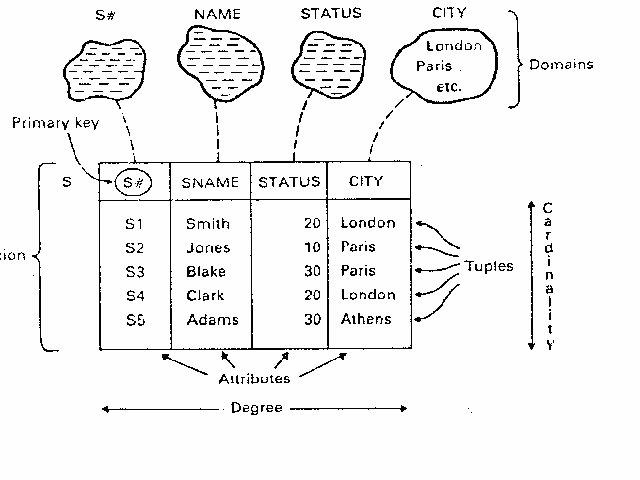# RELATIONAL DATABASES

Click here for audio-text lecture and feed it to the speech agent

Click here for an audio lecture that can be played using RealPlayer
• A relational database is a collection of time-varying, normalized relations of assorted degrees.

• The following intiutitve correspondence can be made:

1. A relation is a file
2. Each file contains only one record type
3. The records have no particular order
4. Every field is single-valued
5. The records have a unique identifying field or composite field, called the primary key field.

• A relational database consists of a collection of tables.
• All data values are atomic. No repeating groups are allowed.
• A relational database is a pointerless database, User does not see or is made aware of pointers.• Relation
• Tuple
• Attribute
• Cardinality
• Degree
• Primary key
• Domain

# Equivalent Database Concepts

• Relation <-> Table
• Tuple <-> Row or record
• Attribute <-> Column or field
• Cardinality <-> Number of rows
• Degree <-> Number of columns
• Primary key <-> Unique identifier
• Domain <-> Pool of legal values

# DOMAINS

• A domain is a named set of scalar values, all of the same type.
Example 1: Domain of P# is the set of character strings of length 6.
Example 2: Domain of WEIGHT is the set of small integers less than 10,000.
Example 3: Domain of QTY is the set of integers less than one billion.
• Therefore, a "domain" is a "data type".

# DOMAINS

• Domains (or data types) can be used to impose semantic constraints.
Example 1:
SELECT P.*, SP.*
FROM P, SP
WHERE P.P# = SP.P# ;
The comparison in the conditional clause is sensible.

Example 2:
SELECT P.*, SP.*
FROM P, SP
WHERE P.WEIGHT = SP.QTY ;
The comparision involves two attributes of different types, and therefore should not be allowed.

# RELATIONS

• A relation R on a collection of domains D1, D2, ..., Dn, consists of two parts: a "heading" and a "body".
• The heading consists of a fixed set of attribute-domain pairs,
{ (A1:D1),(A2:D2), ... , (An,Dn) }
• The heading is also called the schema.
• The body consists of a time-varying set of tuples, where each tuple consists of a set of attribute-value pairs,
{ (A1:vi1), (A2:vi2),..., (An:vin) }
and i = 1, 2, ..., m.
• The body is also called the instance.

# RELATIONS

• In the above, n is the degree of the relation and m is the cardinality of the relation.

• A relation R can be considered as a variable.
• The heading of a relation is the "type" of the variable R.
• The type of R is (D1,D2,...,Dn).
• The body of a relation is the "value" of the variable R.
• The value of R is a subset of the Cartesian Product of D1, D2, ..., Dn.

# AN EXAMPLE OF A RELATION

• The heading of R is (S#, SNAME, STATUS, CITY )
• This is a shorthand notation for { (S#:S#), (SNAME:NAME), (STATUS:STATUS), (CITY,CITY) }
• The body of R is a subset of the product of the underlying domains.
• A typical tuple is an element of this product of domains.
( S1, Smith, 20, London )
• This is a shorthand notation for { (S#: S1), (SNAME: SMith), (STATUS: 20), (CITY: London) }

# CARTESIAN PRODUCT OF DOMAINS

• Suppose the domain of S# is {1,2,3} and the domain of NAME is {Smith, Doe}
• The Cartesian Product of the two domains is denoted by
S# x NAME
• The Cartesian Product is:
{(1,Smith), (1, Doe), (2,Smith), (2,Doe), (3,Smith), (3, Doe) }
• Later we will use the Cartesian Product to explain "equijoin" and "natural join".

# PROPERTIES OF RELATIONS

• P1: There are no duplicate tuples.
• P2: Tuples are unordered.
• P3: Attributes are unordered.
• P4: All attribute values are atomic.

*Notice the implementation of a relational database system often deviates from the above properties (which ones?).

# KINDS OF RELATIONS

• Base relations: The real relations. Called "base table" in SQL.
• Views: The virtual relations. A view is a named, derived relation.
• Snapshots: A snapshot is a real, not virtual, named derived relation.
• Query results: The final output relation from a specified query. It may not be named and has no permanent existence.
• Temporary relations: A nonpermanent named derived relation.